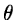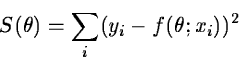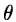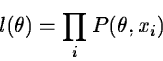Next: Raw to Calibrated Data Up: Basic Concepts Previous: Noise distributions

Estimation

A number of different statistical methods are used for estimating parameters from a data set. The most commonly used one is the least squares method which estimates a parameterby minimizing the function :(2.6)

where y is the dependent and x the independent variables while f is a given function. Equation 2.6 can be expanded to more parameters if needed. For linear functions f an analytic solution can be derived whereas an iteration scheme must be applied for most non-linear cases. Several conditions must be fulfilled for the method to give a reliable estimate of. The most important assumptions are that the errors in the dependent variable are normal distributed, the variance is homogeneous, and the independent variables have no errors and are uncorrelated.

The other main technique for parameter estimation is the maximum likelihood method where the joint probability of the parameter:(2.7)

is maximized. In Equation 2.7, P denotes the probability density of the individual data sets. Normally, the logarithm likelihoodis used to simplify the maximization procedure. This method can be used for any given distribution. For a normal distribution the two methods will give the same result.Next: Raw to Calibrated Data Up: Basic Concepts Previous: Noise distributions
Petra Nass
1999-06-15